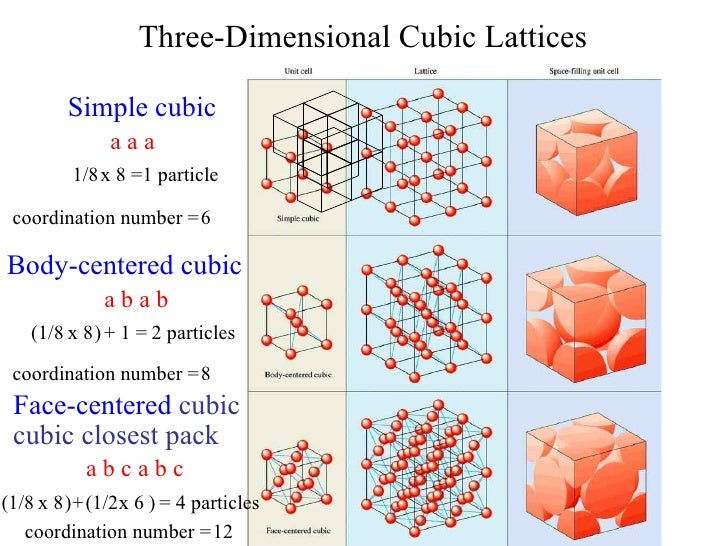# Properties of solid, liquid and gas

## Solid

Types of crystals (solid structure)

1. Metal (metallic crystal) - composed of metal atoms such as Na, Fe and Cu.

2. Ionic structure (ionic crystal) - composed of + and - ions

3. Simple molecule (molecular crystal) - structure formed from small covalent compund such as C02.

4. Covalent crystal - 3D network of covalent bond between atom such as in C (diamond) and silica (SiO2) and quartz.

1. Metallic crystal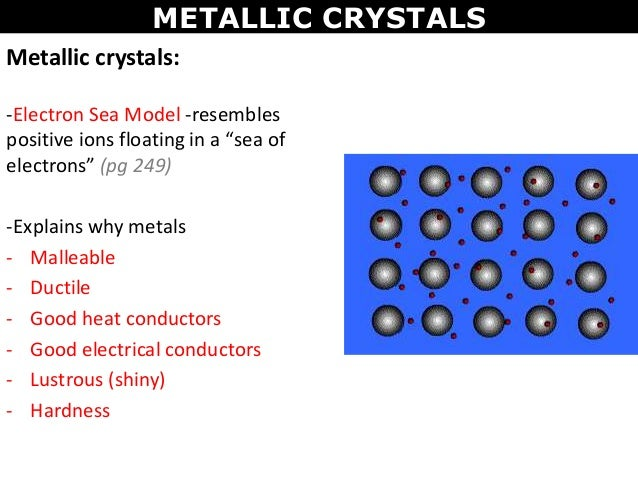1. Have cations at the lattice sites surrounded by mobile electrons.

2. Metallic crystals conduct heat and electricity well, have metallic luster, and tend to have high melting points.

r

have cations at the lattice sites surrounded by mobile electrons.Metallic crystals conduct heat and electricity well, have metallic luster, and tend to have high melting points.

Covalent crystal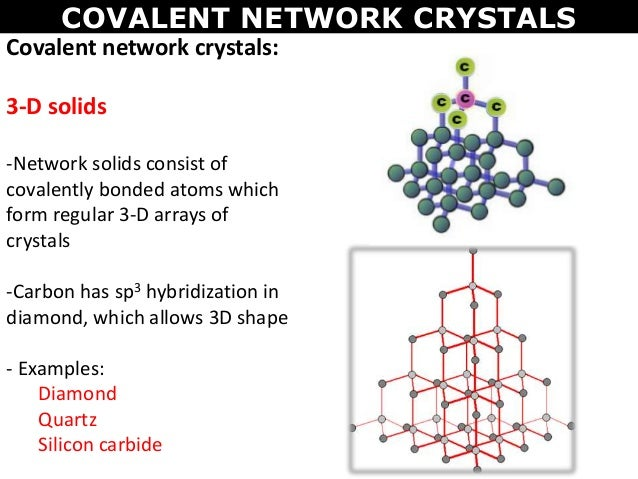1. Have atoms at the lattice sites which is covalently bonded to other atoms.

2. These are also called as network solids and the crystal is essentially one large molecule.

3. A typical example is diamond (very hard, has a very high melting point, and is a nonconductor of electricity).

r

1. Have atoms at the lattice sites covalently bonded to other atoms.2. These are also called network solids and the crystal is essentially one large molecule. A typical example is diamond which is very hard, has a very high melting point, and is a nonconductor of electricity

Ionic crystal

1. Have cations and anions at the lattice sites.

2. Characteristics:

* Brittle
* Have high melting points
* Nonconducting in the solid phase and conducting in the liquid phase.

r

1. Have cations and anions at the lattice sites.2. Characteristics:* Brittle* Have high melting points* Nonconducting in the solid phase and conducting in the liquid phase.

Molecular crystal

1. Have neutral molecules at the lattice sites.

2. Due to the relatively weak intermolecular attractions, solids made from small molecules:

- tend to be soft
- low melting points
- They are nonconducting in both the liquid and solid phase.

r

1. Have neutral molecules at the lattice sites.2. Due to the relatively weak intermolecular attractions, solids made from small molecules:- tend to be soft - low melting points- They are nonconducting in both the liquid and solid phase.

Arrangement of units in crystal

Unit cell : The smallest 3-dimensional unit that describes the arrangement of atoms.

Coordination number :The number of nearest neighbours that surrounds an atom in a crystal.

* In a crystal, they are packed to minimize the space they occupy.1. Crystal lattice : Arrangement of atoms, or groups of atoms, in a crystal. These atoms or groups of atoms are commonly referred to as points within a crystal lattice site. Thus, think of a crystal lattice site as containing a series of points arranged in a specific pattern with high symmetry.

2. Types of unit cell :

* Simple-centered cubic
* Body-centered cubic
* Face-centered cubic

Simple-centered cubic (SCC)

1. The simplest and most symmetrical three- dimensional lattice.

2. Only 1/8 of each atom lies in a particular simple cubic unit cell

Body-centerd cubic (BCC)1. Lattice points in a bcc unit cell are located at each of the eight corners and in the center of the unit cell. A bcc unit cell contains 2 atoms.

2. 2 atoms consist of:

1/8 x 8 = 1 atom
Atom in center = 1 atom

Face-centered cubic (FCC)1. Lattice points in a FCC unit cell are found at each of the eight corners and in the center of each face.

2. A FCC unit cell contains 4 atoms which consists of:

1 atom from the eight corners

1/2 X 6 =3 atoms from each face.

Different types of packing

Close-packed structure : Structures that achieve the maximum packing density.

* The above arrangement resulted in two types of holes: tetrahedral hole and octahedral hole.If the next layer is placed so that the tetrahedral holes are covered, the arrangement is called ' hexagonal close packing' (ABABAB) - HCPIf octahedral holes are covered, it is called 'cubic close packing' (ABCABCABCABC) - CCP

The relationship between edge length and radius of atom* For SCC : a = 2r

* For BCC : a = 4r/square root of 3

* For FCC : a = square root of 8 r

## Gas

Properties

Parameters

1. Volume (V),
2. Temperature (T),
3. Pressure (P) (barometer dan manometer)
4. Amount of gas (for example mol, n).

These parameters are inter related by mathematical equations called gas laws.

Boyle's Law

Temperature constant:

P₁V₁ = P₂V₂

Volume of certain amount of gas ∝
1 / pressure

r

Temperature constant:P₁V₁ = P₂V₂Volume of certain amount of gas ∝ 1 / pressure* Graph shows the relationship between pressure and volume which is inversely-proportional to each other. * The hypothetical gas that does exactly obey Boyle’s law is called an ideal gas. REAL GAS

Gay-Lussacs' Law

Volume constant:

P₁ = P₂
T₁ T₂ P ∝ T

Gay-Lussacs’ law states that:

The pressure of a fixed amount of gas held at constant volume is directly proportional to the temperature (Kelvin, K)

r

Volume constant:P₁ = P₂T₁ T₂Gay-Lussacs’ law states that:The pressure of a fixed amount of gas held at constant volume is directly proportional to the temperature (Kelvin, K)*Graph above shows that pressure is directly proportional to the temperature in Kelvin.

r

*Graph above shows that pressure is directly proportional to the temperature in Kelvin.

Charle's Law

Pressure constant:

V₁ = V₂ V ∝ T
T₁ T₂

The volume of an amount of gas is directly proportional to its temperature (in Kelvin).

r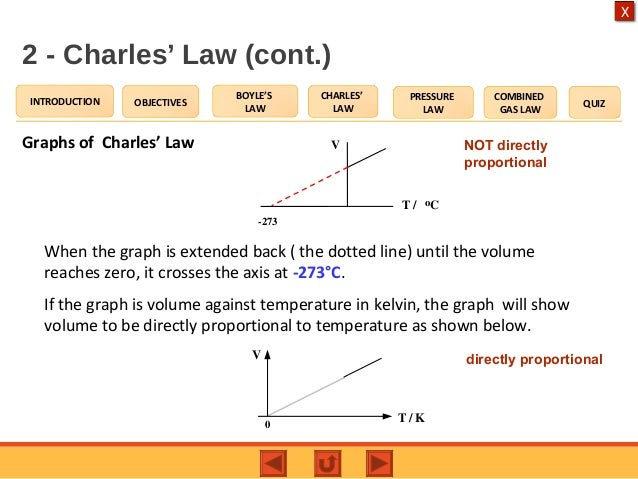* Based on the graph, at -273ºC the gas is (theoretically) zero. This temperature is known absolute zero (0 K).

r

Based on the graph, at -273ºC the gas is (theoretically) zero. This temperature is known asabsolute zero (0 K)

Its about all gases with the same volume has the same number of molecule (mole) at the same T and P.

1. The volume of 1 mol of gas is called molar volume.

2. At STP (0ᵒC, 1 atm), the molar volume of any gas is 22.4 L (or 22.4 dm3)

r

Its about all gases with the same volume has the same number of molecule (mole) at the same T and P.”1. The volume of 1 mol of gas is called molar volume.2. At STP (0ᵒC, 1 atm), the molar volume of any gas is 22.4 L (or 22.4 dm3)

Dalton's Law of Partial PressureGraham's Law : diffusion and effusion

Diffusion - The rate at which two gases mix.

Effusion - The rate at which a gas escapes through a pinhole into a vacuum.

The rate of diffusion/effusion is inversely proportional to the square root of its molar mass under identical conditions of temperature and pressure.

* If two bodies of different masses have the same kinetic energy, the lighter body moves faster!

r

Diffusion - The rate at which two gases mix. Effusion - The rate at which a gas escapes through a pinhole into a vacuum.The rate of diffusion/effusion is inversely proportional to the square root of its molar mass under identical conditions of temperature and pressure. * If two bodies of different masses have the same kinetic energy, the lighter body moves faster!The formula

Kinetic Molecular Theory

* Video above shows some explanations on movement of gases.

Real gas exhibit non-ideal behaviour gas.## Liquid

r

Properties can be understood in terms of how tightly the molecules are packed together and the strength of the intermolecular attractions between them.Subtopic

* Properties can be understood based on how tightly the molecules are packed together and the strength of the intermolecular attractions between them.

Intermolecular forces VS Intramolecular forces

Intramolecular forces - chemical bonds
within molecule.

* Control the chemical properties of substance

Intermolecular forces - the attractions between molecules.

* Control the physical properties of the substance.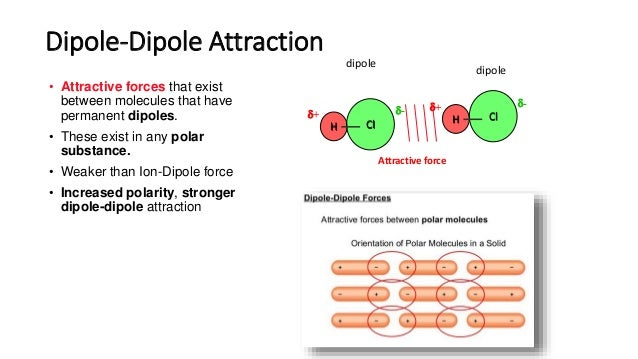Hydrogen bonds

1. Very strong dipole-dipol
e attraction that occur when H is covalently bonded to a small, highly electronegative atom (usually F, O, or N)

* Most common example: H20 m
olecules (tetrahedral 109.5 degree electronic geometry)

2. Typically about ten times stronger than other dipole-dipole attractions. STRONGEST BOND.

3. Responsible for the expansion of water as it freezes.

r

1. Very strong dipole-dipole attraction that occur when H is covalently bonded to a small, highly electronegative atom (usually F, O, or N)2. Typically about ten times stronger than other dipole-dipole attractions. STRONGEST BOND.3. Are responsible for the expansion of water as it freezes.London- dispersion forces:

1. A very weak attraction between non-polar molecules.

2. Arise from the interactions of instantaneous dipoles on neighboring molecules.

r

1. A very weak attraction between non-polar molecules.2. Arise from the interactions of instantaneous dipoles on neighboring molecules.

Ion-dipole:

1. Occur when ions interact with polar molecules

2. It can lead to large net of attractions.

Ion-induced dipole:

1. Occur when an ion induces a dipole on neighboring particle.

2. It depends on ion charge and the polarizability of its neighbour.

General properties of liquid

Volume and shape

r

1. Attraction force between the molecules in liquid basically limit their movement.2. This is the reason why liquid has certain volume but no definite shape * Liquid keeps its volume regardless of the size of container but its shape change depending on the container used.

1. Attraction force between the molecules in liquid basically limit their movement.

2. This is the reason why liquid has certain volume but no definite shape.

* Liquid keeps its volume regardless of the size of container but its shape change depending on the container used.

Compressibility and expansion

1. Liquid is almost incrompressible. DUE TO VERY LITTLE SPACE BETWEEN PARTICLES.

*Have little effect on temperature and pressure

Compressibilty -measure of the ability of a substance to be forced into a smaller volume.

r

1. Defined as the resistance to changing the form of a sample. 2. Factors affecting viscosity:# Intermolecular forces# Long chain molecule# Higher temperature, lower down the viscosity

Surface tension1. Surface tension defined as the tendency of a liquid to take a shape with minimum surface area.

2. Example : water droplets on oily surfaces.

* LIQUID WITH STRONG INTERMOLECULAR ATTRACTIONS, HAVE HIGH SURFACE TENSION.

Wetting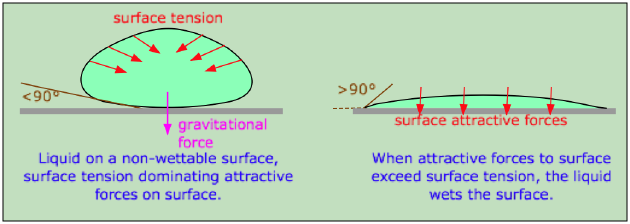1. Ability of water to wet things depend on its cohesive and adhesive forms.

2. Better when adhesive force is higher than cohesive - Example : Clean surface compared to oily surface.

3. Detergent is used to reduce surface tension with the help of surfactants which allow the detergent solution to penetrate into the fabric.

r

1. Defined as the spreading of a liquid across a surface to form a thin film.2.

Viscosity

1. Defined as the resistance to change a form of sample.

2. Factors affecting viscosity:

# Intermolecular forces
# Long chain molecule
# Higher temperature, lower viscosity

Evaporation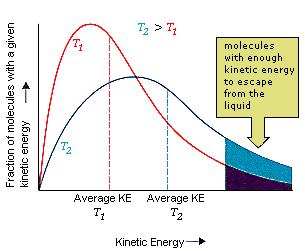Phase transition from liquid to gas.

* Molecules that are able to escape from the liquid have kinetic energies larger than the average. When they leave, the average kinetic energy of the remaining molecules is less, so the temperature is lower.

* Higher temperature, higher rate of evaporation.

-

SublimationPhase transition from solid to gas phase without going through liquid phase.

- Triple point : All three phases are in equilibrium.

- Critical point : Above this point, it is impossible to condense a gas into a liquid just by increasing the pressure.

## 1. From Boyle’s and Charles’s Law: V is proportional to T/P PV/T = k (constant)2. For 2 state of the same number of mole:P₁V₁ = P₂V₂ T₁ T₂3. When combined with Avogadro’s principle: V proportional to nT/P PV/nT = constant = R or PV = nRTThis is known as Ideal Gas Law: R = gas constant = 0.082 atm L mol-1 K-1 = 8.31 Nm K-1 mol-1 = 8.31 J K-1 mol-1

r

1. From Boyle’s and Charles’s Law, V is proportional to T/P PV/T = k (constant)2. For 2 state of the same number of mole: P₁V₁ = P₂V₂ T₁ T₂ 3. When combined with Avogadro’s principle: V proportional to nT/P PV/nT = constant = R or PV = nRTThis is known as Ideal Gas Law R = gas constant = 0.082 atm L mol-1 K-1 = 8.31 Nm K-1 mol-1 = 8.31 J K-1 mol-1

## Always stronger than